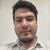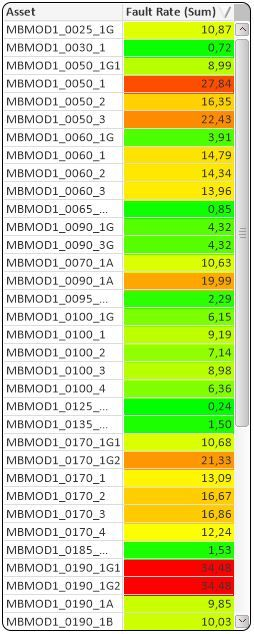# QlikView App Dev

Discussion Board for collaboration related to QlikView App Development.

Announcements
Now Live: Qlik Sense SaaS Simplified Authoring – Analytics Creation for Everyone: READ DETAILS
cancel
Showing results for
Did you mean:Contributor II

## Problem in Sort a straight table after use ColorMix2 in background of an expression! ** With File **

Dearl all,

I'm with a big problem (at least for me, rs*) where I need to use a heatmap with colors red, yellow and  green, in a table sorted from highest to lowest.
The problem is, when I use the function 'top' inside the ColorMix2 the sort function is disable. So far so good, what I'm having trouble is finding a code that replaces this one:

ColorMix2 (if(rangemin(RangeMax (top(total Sum(Fator_FR),1,NoOfRows(total))),rangemax(Sum(Fator_FR),RangeMin (top(total Sum(Fator_FR),1,NoOfRows(total)))))<RangeAvg (top(total Sum(Fator_FR),1,NoOfRows(total))),(rangemin(RangeMax (top(total Sum(Fator_FR),1,NoOfRows(total))),rangemax(Sum(Fator_FR),RangeMin (top(total Sum(Fator_FR),1,NoOfRows(total)))))-RangeAvg (top(total Sum(Fator_FR),1,NoOfRows(total))))/(RangeAvg (top(total Sum(Fator_FR),1,NoOfRows(total)))-RangeMin (top(total Sum(Fator_FR),1,NoOfRows(total)))),(rangemin(RangeMax (top(total Sum(Fator_FR),1,NoOfRows(total))),rangemax(Sum(Fator_FR),RangeMin (top(total Sum(Fator_FR),1,NoOfRows(total)))))-RangeAvg (top(total Sum(Fator_FR),1,NoOfRows(total))))/(RangeMax (top(total Sum(Fator_FR),1,NoOfRows(total)))-RangeAvg (top(total Sum(Fator_FR),1,NoOfRows(total))))), ARGB(255, 0, 255, 0), ARGB(255, 255, 0, 0), ARGB(255, 255, 255, 0))There is any other code that I can use  instead of this to get the same (or similar) heatmap result  and can sort the expression column from highest to lowest?

Thanks for everyone's help!

Labels (3)

• ### top